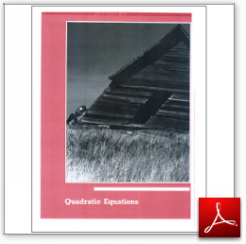Algebra Math Book - Introductory Algebra Thank you for your continual support.

 The power of mathematics rests in the logic of thinking.ID: Chapter10
Price: 9.95

# Algebra Math book - Introductory Algebra - Chapter 10 - Quadratic Equations

## Chapter 10 of this algebra math book has 5 sections.

To get started, you might like to take a free test just to see what areas you might need to study.  Take the test.

 Sec10.1 Section10.1-General Form of a Quadratic Equation Sec10.2 Section10.2-Solving Quadratic Equations by Factoring Sec10.3 Section10.3-Completing the Square Sec10.4 Section10.4-Quadratic Formula Sec10.5 Section10.5-Applied Problems

For a complete listing of the objectives in this chapter visit the List of Objectives.

This section of my algebra math book, Introductory Algebra, also includes in the download:

• Cover Sheet